Study Materials: ncert solutions

Our ncert solutions for Exercise 2.3 Class 10 maths 2. Polynomials - ncert solutions - Toppers Study is the best material for English Medium students cbse board and other state boards students.

Solutions ⇒ Class 10th ⇒ Mathematics ⇒ 2. Polynomials

# Exercise 2.3 Class 10 maths 2. Polynomials - ncert solutions - Toppers Study

Topper Study classes prepares ncert solutions on practical base problems and comes out with the best result that helps the students and teachers as well as tutors and so many ecademic coaching classes that they need in practical life. Our ncert solutions for Exercise 2.3 Class 10 maths 2. Polynomials - ncert solutions - Toppers Study is the best material for English Medium students cbse board and other state boards students.

## Exercise 2.3 Class 10 maths 2. Polynomials - ncert solutions - Toppers Study

CBSE board students who preparing for class 10 ncert solutions maths and Mathematics solved exercise chapter 2. Polynomials available and this helps in upcoming exams 2023-2024.

### You can Find Mathematics solution Class 10 Chapter 2. Polynomials

• All Chapter review quick revision notes for chapter 2. Polynomials Class 10
• NCERT Solutions And Textual questions Answers Class 10 Mathematics
• Extra NCERT Book questions Answers Class 10 Mathematics
• Importatnt key points with additional Assignment and questions bank solved.

NCERT Solutions do not only help you to cover your syllabus but also will give to textual support in exams 2023-2024 to complete exercise 2.3 maths class 10 chapter 2 in english medium. So revise and practice these all cbse study materials like class 10 maths chapter 2.3 in english ncert book. Also ensure to repractice all syllabus within time or before board exams for ncert class 10 maths ex 2.3 in english.

See all solutions for class 10 maths chapter 2 exercise 2 in english medium solved questions with answers.

### Exercise 2.3 class 10 Mathematics Chapter 2. Polynomials

• Exercise 2.3 Class 10 Maths 2. Polynomials - Ncert Solutions - Toppers Study
• Class 10 Ncert Solutions
• Solution Chapter 2. Polynomials Class 10
• Solutions Class 10
• Chapter 2. Polynomials Exercise 2.3 Class 10

## Exercise 2.3 Class 10 maths 2. Polynomials - ncert solutions - Toppers Study

### Exercise 2.3 class 10 maths chapter 2. Polynomials

Q1. Divide the polynomial p(x) by the polynomial g(x) and find the quotient and remainder in each of the following:

(i) p(x) = x3 – 3x2 + 5x – 3, g(x) = x2 – 2

(ii) p(x) = x4 – 3x2 + 4x + 5, g(x) = x2 + 1 – x

(iii) p(x) = x4 – 5x + 6, g(x) = 2 – x2

Solution: (i) p(x) = x3 – 3x2 + 5x – 3, g(x) = x2 – 2Quotients q(x) = x –  3 and Remainder = 7x –  9

Solution: (ii) p(x) = x4 – 3x2 + 4x + 5, g(x) = x2 + 1 – x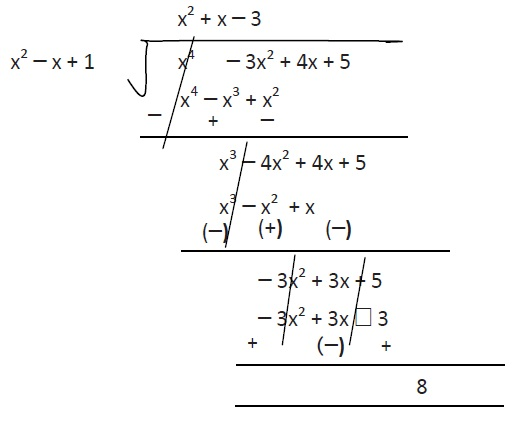Quotients q(x) = x2 + x –  3 and Remainder = 8

Solution: (iii) p(x) = x4 – 5x + 6, g(x) = 2 – x2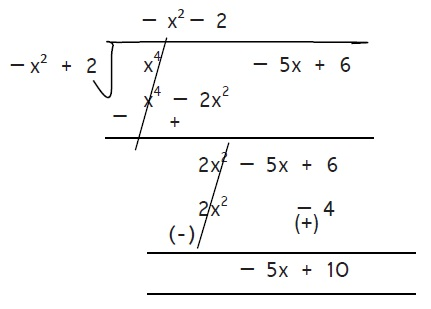Quotients q(x) = – x2 –  2 and Remainder = –  5x + 10

Q2. Check whether the first polynomial is a factor of the second polynomial by dividing the second polynomial by the first polynomial:

(i) t2 – 3, 2t4 + 3t3 – 2t2 – 9t – 12

(ii) x2 + 3x + 1, 3x4 + 5x3 – 7x2 + 2x + 2

(iii) x3 – 3x + 1, x5 – 4x3 + x2 + 3x + 1

Solution: (i) t2 – 3, 2t4 + 3t3 – 2t2 – 9t – 12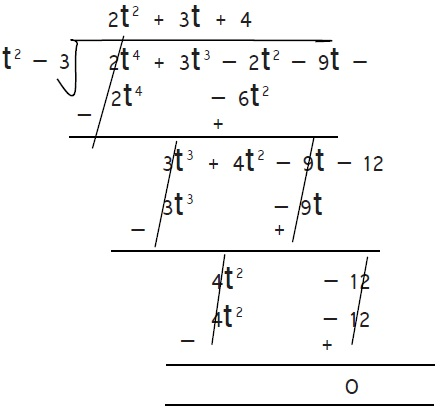Hence Remainder r(x) is 0

Therefore, t2 – 3 is the factor of 2t4 + 3t3 – 2t2 – 9t – 12

Solution: (ii) x2 + 3x + 1, 3x4 + 5x3 – 7x2 + 2x + 2Hence Remainder r(x) is 0

Therefore,  x2 + 3x + 1 is the factor of 3x4 + 5x3 – 7x2 + 2x + 2

Solution: (iii) x3 – 3x + 1, x5 – 4x3 + x2 + 3x + 1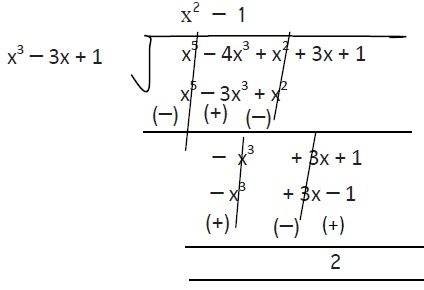Hence Remainder r(x) = 2

Therefore, x3 – 3x + 1, is not a factor of x5 – 4x3 + x2 + 3x + 1

Q3. Obtain all other zeroes of 3x4 + 6x3 – 2x2 – 10– 5, if two of its zeroes areSolution:

Given that : p(x) = 3x4 + 6x3 – 2x2 – 10– 5Or 3x2​ - 5 = 0

Therefore, 3x2 - 5 is the factor of p(x)

Now Dividing 3x4 + 6x3 - 2x2 - 10x - 5 by 3x2 - 5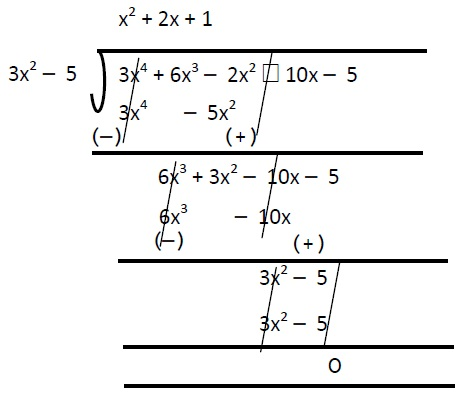Therefore,  p(x) = (3x2 –  5) (x2 + 2x + 1)

Now, factorizing and getting zeroes x2 + 2x + 1 -

= x2 + x + x + 1 = 0

= x(x + 1) + 1(x + 1) = 0

= (x + 1) (x + 1) = 0

Or x + 1 = 0, x + 1 = 0

Or x = – 1, x = – 1

Therefore, two zeroes are – 1 and – 1.

Q4. On dividing x3 – 3x2 + + 2 by a polynomial g(x), the quotient and remainder were – 2 and –2+ 4, respectively. Find g(x).

Solution:

Given that: Dividend p(x) = x3 – 3x2 + x + 2

Quotient q(x) = x – 2,

Remainder r(x) = – 2x + 4

Divisor g(x) =?

Dividend = divisor × quotient + remainder

p(x) = g(x) × q(x) + r(x)

x3  3x2 + x + 2 = g(x) (x  2) + ( 2x + 4)

x3  3x2 + x + 2 + 2x  4 = g(x) (x  2)

g(x) (x  2) = x3  3x2 + 3x  2Dividing x3  3x2 + 3x  2 by x - 2 we obtain g(x)-Therefore, Divisor g(x) = x2  x + 1

Q5. Give examples of polynomials p(x), g(x), q(x) and r(x), which satisfy the division algorithm and

(i) deg p(x) = deg q(x)

(ii) deg q(x) = deg r(x)

(iii) deg r(x) = 0

Solution:

Using Euclid’s Division algorithm:

p(x) = g(x) × q(x) + r(x)  where q(x)  0

(i) deg p(x) = deg q(x)

The deg of dividend p(x) and quotient q(x) can be equal when deg of divisor is 0 or any number.

Example : Let p(x) = 2x2 - 6x + 3

And let g(x) = 2

On dividing

p(x) = 2x2 - 6x + 2 + 1

= 2(x2 - 3x + 1) + 1

Now comparing 2(x2 - 3x + 1) + 1 by p(x) = g(x) × q(x) + r(x) we get:

So, q(x) = x2 - 3x + 1and r(x) = 1

By which we obtain deg p(x) = deg q(x)

(ii) deg q(x) = deg r(x)

Solution: This situation comes when deg p(x) and deg g(x) is equal-

Let p(x) = 2x2 + 6x + 7 and g(x) = x2 + 3x + 2

On dividing: q(x) = 2 and r(x) = 3

Therefore, deg q(x) = deg r(x)

(iii) deg r(x) = 0

Solution:

r(x) = 0 is obtained when p(x) is completely divisible by g(x):

Let p(x) = x2 – 1 and g(x) = x + 1

On dividing we obtain:

q(x) = x – 1 and r(x) = 0

##### Other Pages of this Chapter: 2. Polynomials

Important Study materials for classes 06, 07, 08,09,10, 11 and 12. Like CBSE Notes, Notes for Science, Notes for maths, Notes for Social Science, Notes for Accountancy, Notes for Economics, Notes for political Science, Noes for History, Notes For Bussiness Study, Physical Educations, Sample Papers, Test Papers, Mock Test Papers, Support Materials and Books.Mathematics Class - 11th

NCERT Maths book for CBSE Students.

books

## Study Materials List:

##### Solutions ⇒ Class 10th ⇒ Mathematics
1. Real Numbers
2. Polynomials
3. Pair of Linear Equations in Two Variables
5. Arithmetic Progressions
6. Triangles
7. Coordinate Geometry
8. Introduction to Trigonometry
9. Some Applications of Trigonometry
10. Circles
11. Constructions
12. Areas Related to Circles
13. Surface Areas and Volumes
14. Statistics
15. Probability

New Books